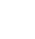# Recreate each worksheet as values instead of formulas

``` 'Recreate each worksheet as values instead of formulas and scroll to top / A1 For Each ws In Worksheets ws.Activate ws.Cells.Select Selection.Copy Selection.PasteSpecial Paste:=xlPasteValues ws.Range("a1").Select Application.Goto Reference:=ws.Range("a1"), Scroll:=True Next ws ```

This VBA can be applied to any workbook where you want the formulas to be copied and pasted as values – ie as text in every worksheet.

Warning, this essentially erases the formulas in the workbook so ensure you have saved a copy if you need to retain the formulas.